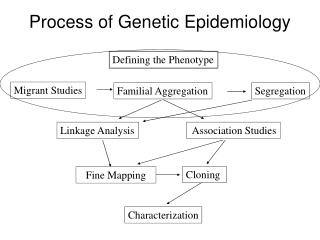Download PresentationProcess of Genetic EpidemiologyProcess of Genetic Epidemiology - PowerPoint PPT Presentation

Download PresentationProcess of Genetic Epidemiology
An Image/Link below is provided (as is) to download presentation

Download Policy: Content on the Website is provided to you AS IS for your information and personal use and may not be sold / licensed / shared on other websites without getting consent from its author. While downloading, if for some reason you are not able to download a presentation, the publisher may have deleted the file from their server.

- - - - - - - - - - - - - - - - - - - - - - - - - - - E N D - - - - - - - - - - - - - - - - - - - - - - - - - - -
Presentation Transcript

1. Process of Genetic Epidemiology Defining the Phenotype Migrant Studies Familial Aggregation Segregation Linkage Analysis Association Studies Cloning Fine Mapping Characterization

2. Familial Aggregation • Does the phenotype tend to run in families?

3. Recurrence (‘Familial’) Risk Ratios • Compares the probability a subject is affected given they have an affected family member to the population risk: lR = KR/K, where KR is the risk to relatives of type R K is the population risk lS = recurrence risk to siblings of probands versus the general population risk.

4. Recurrence Risk Ratios lR = P(Y2 = 1 |Y1 = 1) / K P(Y2=1|Y1=1)P(Y1=1) = P(Y2=1, Y1=1) P(Y2=1|Y1=1) = P(Y2=1, Y1=1)/P(Y1=1) K = P(Y1=1) lR = P(Y2=1, Y1=1)/P(Y1=1)2

5. Estimating RRR • With case-control data, calculate FRR as: Proportion of affected relatives of the cases (observed) / Proportion of affected relatives of controls (expected) (assumed to estimate K) • The higher the value of l, the stronger the genetic effect

6. Examples of s • Alzheimer Disease 3-4 • Rheumatoid Arthritis 12 • Schizophrenia 13 • Type I Diabetes 15 • Multiple Sclerosis 20-30 • Neural Tube Defects 25-50 • Autism 75-150

7. s versus GRR • How well does s estimate the genetic risk ratio? s = P(Y2=1|Y1=1) / P(Y2=1|Y1=0) ? P(Y2=1|D) / P(Y2=1|dd) = GRR Sibs disease not necessarily hereditary At risk individual may not have inherited D Sib unaffected doesn’t mean other sib doesn’t carry D

8. s versus GRR

9. Heritability Analysis • Evaluates the genetic contribution to a trait Y in terms of variance explained. • Y = Genetics + Environment • Var(Y) = overall variation in phenotype Y = Var(G) + Var(E) + 2Cov(G,E) • Broad sense heritability: H2 = Var (G) / Var (Y) where Var(G) = genetic part of variance = VA+VD (Additive + Domince)

10. Narrow Sense Heritability • Proportion of phenotypic variance that is explained only by additive genetic effects: h2= VA/ Var (Y) A number of ways to estimate heritability. Commonly done with twin studies.

11. Twin Studies • Compare the phenotype correlation or disease concordance rates of MZ (identical) and DZ (fraternal) twins. Twin 1

12. MZ Twins (Identical) Twin 1 Both alleles are shared identical by descent (IBD) Twin 2

13. DZ Twins (Fraternal) Twin 1 Twin 2: any of the four IBD can be 2, 1, or 0 2 1 1 0

14. DZ Twins (Fraternal) Twin 1 Average sharing is 50% 100% 50% 50% 0%

15. IBD Sharing # of alleles shared IBD 2 1 0 Pr(2) Pr(1) Pr(0) Prop IBD Relationship Self, MZ twins 1 0 0 1 Parent, Offspring 0 1 0 1/2 Full siblings 1/4 1/2 1/4 1/2 Gr-child, Gr-prt 0 1/4 3/4 1/4 First cousins 0 1/4 3/4 1/8 Proportion of alleles shared IBD = # alleles x Pr(# alleles) / 2

16. Twin Studies • Assume MZ twins share all genes & envt., DZ share ½ genes & all envt. • Correlation among twins: rmz= VA+ VE rdz= ½ VA + VE where VE = common environment H2 = 2(rmz- rdz ) • Heritability ~ two times difference in correlation between MZ and DZ twins.

17. Example of Twin Study: PCa • Twin registry (Sweden, Denmark, and Finland) • 7,231 MZ and 13,769 DZ Twins (male) Heritability: 0.42 (0.29-0.50) Non-shared Environment: 0.58 (0.50-0.67) Lichtenstein et al NEJM 2000 13;343:78-85. Limitations of heritability calculations?

18. Segregation Analysis • Study families. • Estimate ‘mode of inheritance’ & what type of genetic variant might be causal. • Determine whether the disease appears to follow particular patterns across generations. • Estimate whether variants are rare or common, etc.

19. Segregation: Harry Potter’s Pedigree Muggle Wizard / Witch Vernon Dursley Lily Evans James Potter Petunia Dursley Harry Potter Dudley Dursley

20. Segregation Analysis • What is the best model of inheritance for observed families? • Dominant • Recessive • Additive • Disease allele frequency? • Magnitude of risk? • Fit formal genetic models to data on disease phenotypes of family members. • The parameters of the model are generally fitted finding the values that maximize the probability (likelihood) of the observed data. • This information is useful in parametric linkage analysis, which assumes a defined model of inheritance.